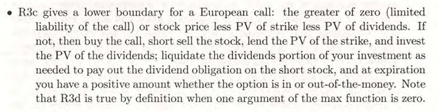# Why is an European call option always greater in value than max(0,S-X*exp(-r(T-t))-D)?This is an excerpt from the book by Timothy Crack. What I don’t understand is how can I always remain cash flow positive. For example, let’s say I short the stock (receive S), I buy the call for let’s say S-Xexp(-r(T-t)) - D -1 (call value less than max), where X = strike price and D = Dividends, then let’s say I am in the money hence I exercise the call and pay X and use the stock to repay my short. Also, I pay dividends to my short and I receive interest on Present value of strike price (Xexp(-r(T-t)) and dividends which I invest, so my cash flow is S-(S-X*exp(-r(T-t)) - D -1) - X - D + interest (on PV of X and D) = X(exp(-r(T-t)) - 1) +1 +interest but if X is large I can be cash flow negative? Please correct me if I am wrong.